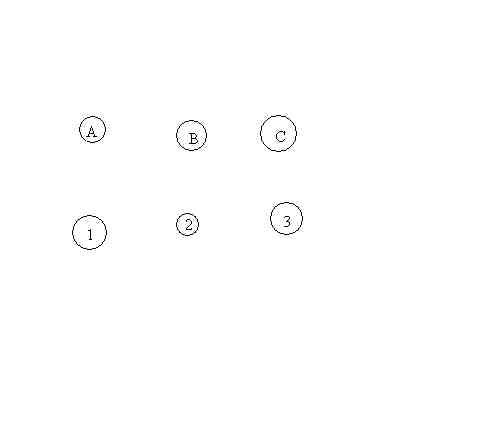# Classification of Platonic Solids

Topological/Graph Theory Proof.
Euclid: "I say next that no other figure, besides the said five figures, can be constructed which is contained by equilateral and equiangular figures equal to one  another."

Proof:
Consider the number of vertices (V>3), edges (E>3), and faces (F>2) involved in the figure.
Since the figure is topologically equivalent to the sphere,

V+F=E+2.(*)
But the assumption is that the number of edges on each face is the same... call it k
and that the number of edges meeting at each vertex is the same... call it l.
Thus we have kF=2E and lV=2E.
Putting this together with (*) we have 2E/l +2E/k = E + 2, or
1/l + 1/k = 1/2 + 1/E.

Here is a table showing the only possible integer values that satisfy this equation and therefore there can be only 5 corresponding regular convex polyhedra- the five platonic solids:

 V F E k l Tetrahedron 4 4 6 3 3 Cube 8 6 12 4 3 Octahedron 6 8 12 3 4 Icosahedron 12 20 30 3 5 Dodecahedron 20 12 30 5 3

# Utility Problem

The classical "utility problem" or "cranky neighbour problem" asks
whether it is possible for three houses (A, B, and C) each to be
connected to three utility plants (1, 2, and 3) without having any of
the lines cross.This problem is impossible to solve in the plane (but not on a torus).
Proofs of the impossibility can be based on the Jordan Curve Theorem or by a counting argument based on Euler's Formula. [V+F=E+2.(*)]

Here is the proof based on Euler's Formula briefly:

If the problem can be solved then it would form a planar graph with V=6 and E=9.
Thus there would have to be exactly 5 regions (including the unbounded region of the plane in the graph).
Each of these regions must have at least 4 edges. Since each edge can be counted twice once for each region it bounds-there will be at least 10 edges in the graph. BUT we only have 9 edges!